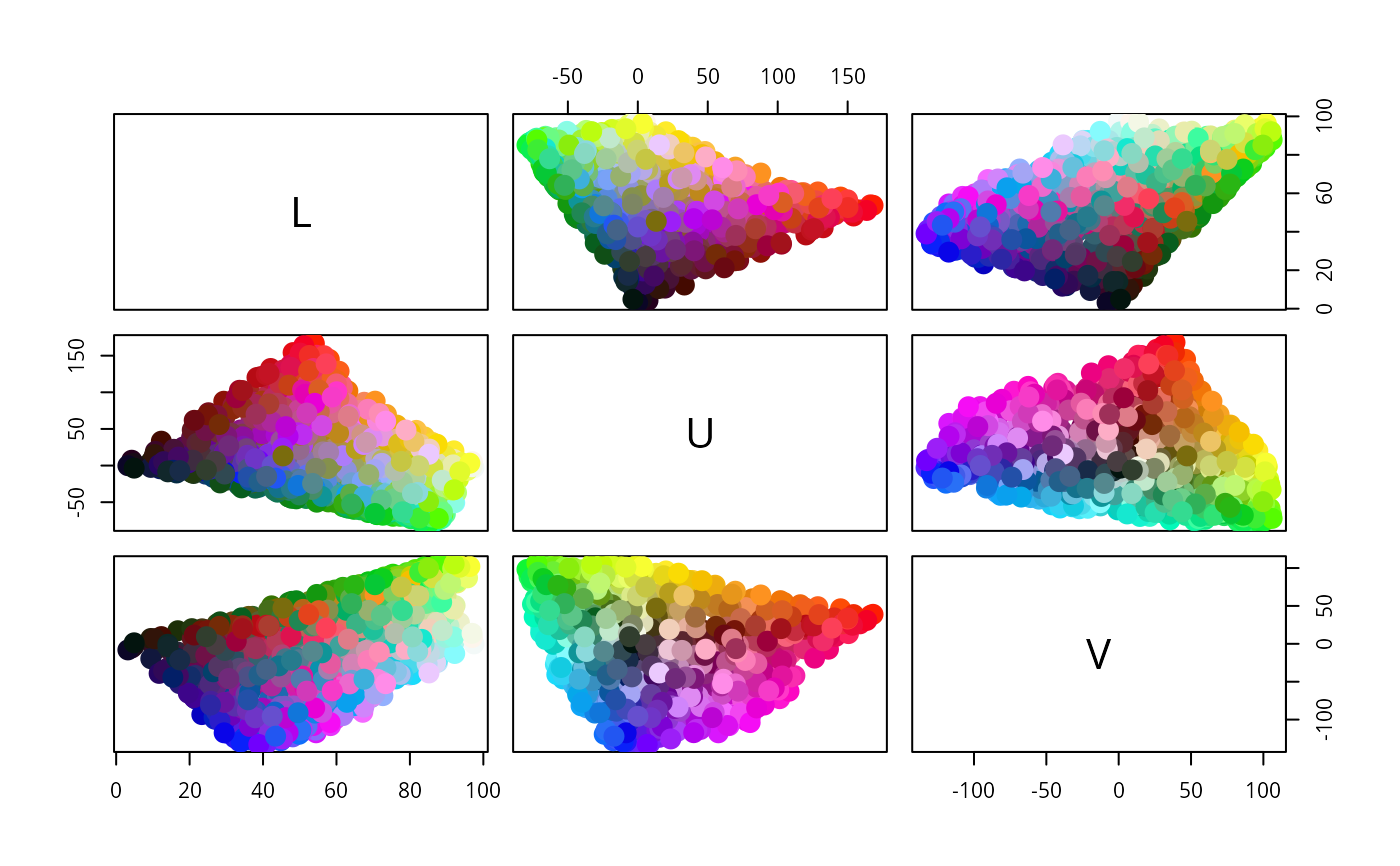This function creates colors of class LUV''; a subclass of the virtual color-class class.

LUV(L, U, V, names)

## Arguments

L, U, V these arguments give the L, U and V coordinates of the colors. The values can be provided in separate L, U and V vectors or in a three-column matrix passed as L. a vector of names for the colors (by default the row names of L are used).

## Value

An object of class LUV which inherits from class color.

## Details

The L, U and V values give the coordinates of the colors in the CIE (1976) $$L^*u^*v^*$$ space. This is a transformation of the 1931 CIE XYZ space which attempts to produce perceptually based axes. Luminance takes values between 0 and 100, and the other coordinates typically take values between -100 and 100, although these values can also be exceeded by highly saturated colors. The $$u$$ and $$v$$ coordinates measure positions on green/red and blue/yellow axes.

RGB, HSV, XYZ, LAB, polarLAB, polarLUV.

Ross Ihaka

## Examples

## Show the LUV space
set.seed(1)
x <- RGB(runif(1000), runif(1000), runif(1000))
y <- as(x, "LUV")